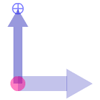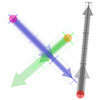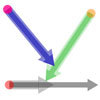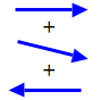# Search by Topic

Filter by: Content type:
Age range:
Challenge level:

### There are 5 results

Broad Topics > Calculus > Complex analysis### Impedance Can Be Complex!

##### Age 16 to 18 Challenge Level:

Put your complex numbers and calculus to the test with this impedance calculation.### Twizzle Arithmetic

##### Age 14 to 16 Challenge Level:

Arrow arithmetic, but with a twist.### Arrow Arithmetic 3

##### Age 14 to 16 Challenge Level:

How can you use twizzles to multiply and divide?### Arrow Arithmetic 2

##### Age 14 to 16 Challenge Level:

Introduces the idea of a twizzle to represent number and asks how one can use this representation to add and subtract geometrically.### Arrow Arithmetic 1

##### Age 14 to 16 Challenge Level:

The first part of an investigation into how to represent numbers using geometric transformations that ultimately leads us to discover numbers not on the number line.